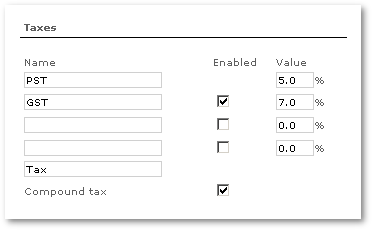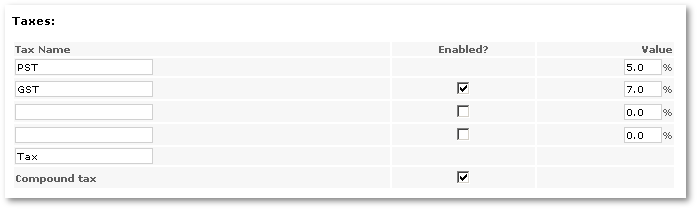# Description

MOR allows you to set several TAXes for Users, Calling Card Groups and Vouchers. All Prices and Rates in MOR are without TAXes. That's a rule.

• Each User has a TAX percent. The User is charged by this TAX percent when he makes Payments or views his Rates.
• Each Calling Card Group has a TAX percent it is applied for each Card of concrete Card Group when Card is Sold.
• Each Voucher has a TAX percent it is applied for each Voucher when it is used.

The Multi-Tax system provides for several TAXes in the final reports for Users, for example - Invoices.

There is two types of Multi-Tax usage:

### Compound Tax

Let's say we have taxes T1, T2, T3 and T4. And some amount without tax, X.

When Compound tax is checked, multiple taxes are calculated in following way:

Amount with Compound Taxes = (((X + T1%) + T2%) + T3%) + T4%

Example

We have initial data:

```SUBTOTAL 3.99
TAX1: 18%
TAX2: 15%
```

Calculating when Compound tax is enabled:

```Amount with Compound tax = (SUBTOTAL + TAX1) + TAX2
```

in digits:

```(SUBTOTAL + TAX1) = 3.99 + 18% = 3.99 + 3.99 / 100 * 18 = 3.99 * 1.18 = 4.7082
```
```(SUBTOTAL + TAX1) + TAX2 = 4.7082 + 15% = 4.7082 + 4.7082 / 100 * 15 = 4.7082 + 0.70623 = 4.7082 * 1.15 = 5.41443
```
```Amount with Compound tax = 5.41443
```

### Simple Tax

Let's say we have taxes T1, T2, T3 and T4. And some amount without tax, X.

If Compound tax is disabled, then:

Amount with Simple Taxes = X + (T1% + T2% + T3% + T4%)

Example

We have initial data:

```SUBTOTAL 3.99
TAX1: 18%
TAX2: 15%
```

Calculating when Compound tax is disabled (Simple tax):

```Amount with Simple tax = SUBTOTAL + (TAX1 + TAX2)
```

in digits:

```SUBTOTAL + (TAX1 + TAX2) = 3.99 + (18% + 15%) = 3.99 + 33% = 3.99 + 3.99 / 100 * 33 = 3.99 + 1.3167 = 3.99 * 1.33 = 5.3067
```
```Amount with Simple tax = 5.3067
```

As an example we can take Canada, which has GST and PST taxes that should be shown on invoices, reports, and so on:

• Open SETTINGS –> Setup –> Settings –> Tax
• Enter Total Tax name (i.e. Tax)
• Check Compound tax
• Enter Tax 1 name (i.e. GST) and value (i.e. 5.0%)
• Check Active? near Tax 2
• Enter Tax2 name (i.e. PST) and value (i.e. 7.0%)
• Click Save changes

Taxes are applied to all newly created Users:and Calling Cards:If you change taxes, you can assign this to all Users/Calling Cards/Vouchers by pressing the buttons below: